Some spherical tilings

 An interesting edge-to-edge tiling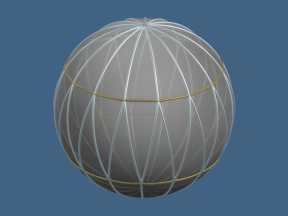This tiling of the sphere with congruent triangles belongs to an infinite family that contains tilings with 12,16,20,... congruent triangles, with 3,4,5,... meeting at each pole. The tiling with five triangles meeting at the pole uses equilateral triangles and is the spherical form of the icosahedron. In either case, omitting the short (gold) edges yields a tiling by spherical rhombi. By rearranging the triangles, we can get the next tiling: A twisted edge-to-edge tiling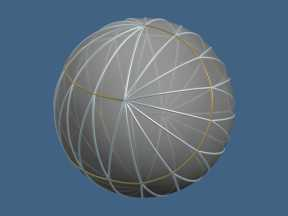This is derived from the previous tiling (in the cases in which there are an odd number of triangles meeting at the pole), which uses the same shape and number of tiles. (On the sphere, two triangles with the same shape must be the same size, as angular excess determines area! Half the triangles have been rotated through 120° with respect to the other half. This "baseball" tiling has a very small symmetry group, but it is not (as we have seen) forced by the shape of the tile. The icosahedron is a special case of this tiling too. Again, omitting the short edges yields a tiling by rhombi. There are, by the way, completely asymmetric tilings of the sphere with triangles. However, at this point (June 2001) no triangle is known that tiles the sphere only with trivial symmetry group. Semilune and quarterlune tilings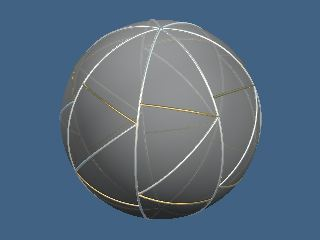These tilings are created by first dividing the sphere into congruent lunes, then either dividing the lunes into two (silver only) or four (both silver and gold edges). For any shape of tile there are many tilings, as each lune can be individually flipped. If the number of triangles meeting at the pole is odd, the isosceles semilune must tile in lune pairs and cannot tile edge-to-edge. If the number is even, the situation is more complicated. There are edge-to-edge and non-edge-to-edge tilings; there are continuum families in which two individually tiles hemispheres can rotate against each other; and there are swirl tilings in which the space between two convex polar caps is filled with lunes. Swirl lune tilings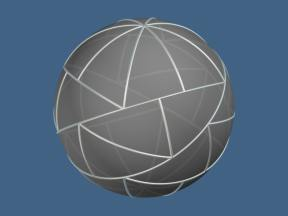These tilings are created from more regular tilings whose tiles can be grouped into lunes. Provided that certain angle conditions are met, a large number of these swirl tilings can be generated from one shape of tile. One or more lunes are set aside and dissected into tiles, which are rearranged into two congruent convex polar caps. The remaining lunes are used to fill the gap between the caps. A tiling from an infinite family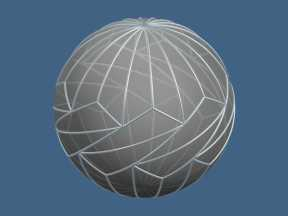This tiling is part of an infinite family whose members have 6,8,10,... triangles meeting at each pole (and 18,24,30,... triangles in all.) The first tiling in the sequence is combinatorially different because its rosettes cross the equator and touch each other. The second tiling (with 24 tiles) is edge-to-edge, with 60°-60°-120° triangles. The special case: 120°-60°-40°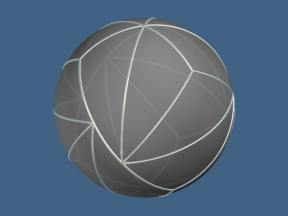This is a special case of the infinite family of tilings above in which the polar rosettes are so large that the tips cross the equator, separating the "filler" into three distict quadrilaterals. We can divide the 120°-60°-40° triangle into four 90°-60°-40° triangles; these also tile as halves of the 80°-60°-60° triangle. The 30°-45°-120° tiling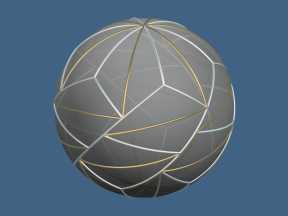This is a "sporadic" tiling that does not fit into any infinite families. If you omit the gold edges, you obtain a tiling by 24 kites. The complicated equatorial belt makes this especially elegant. Like the 90°-72°-30° tiling below, this tiling has four distinct vertex configurations. This explains the lack of variants with more or fewer triangles at the pole. Even without the vertex equation given by the polar configuration, there are three independent linear equations satisfied by the angles, which are therefore completely determined. The 80°-60°-60° tiling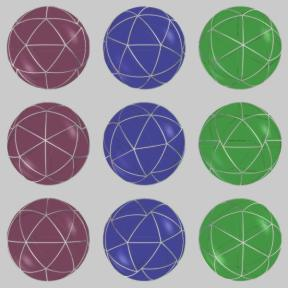The picture shows the three ways (and there are exactly three ) in which the 80°-60°-60° triangle can tile the sphere. The three rows are Northern, equatorial, and Southern views of thesame three tilings. The tiling shown in green is the most symmetric; it has a 12-element symmetry group including (which is unusual among non-edge-to-edge tilings) some mirror symmetries. The tiling is most easily thought of as made up from six six-triangle kites (top and center of the middle image); three of these make up a star at the North Pole, three at the South. The other two have smaller symmetry groups; the tiling at the left has a symmety group generated by two reflections, that in the middle has a symmetry group of order two, generated by a 180° rotation. None of these tilings fit into any infinite families. However, bisecting the tile yields the 90°-60°-40° triangle , which of tiles the sphere in even more ways. This tile, the isosceles semilunes with small angle 360°/(2n+1), and the tiles in the next two tilings are the only isosceles triangles that tile but cannot do so in an edge-to-edge way. The 60°-60°-100° tiling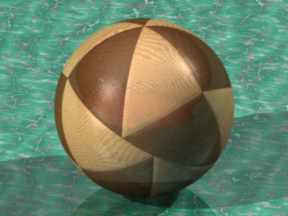This tile tiles only in this way. It is easily shown that the only way to use 100° angles is to put three of them and a 60° angle around a point; this forces a six-triangle "supertile", three of which tile the sphere. It may be bisected to yield the 90°-60°-50° triangle, which tiles the sphere; and it is a special case of an infinite family of tilings in which 2,3,4,... six-triangle supertiles cover the sphere. When n=2 the tiling is edge-to-edge. Like this tiling, and unlike most non-edge-to-edge tilings, they are all two-colorable. Another infinite family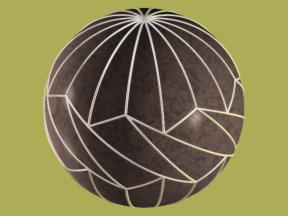These tilings are the infinite family to which the previous 60°-60°-100° tiling belongs. The 60°-60°-150° tiling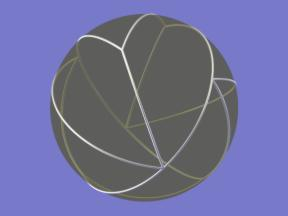This tile tiles only in this way. The 150° angles can only be used up by putting two of them and one 60° angle around a point, which forces a four-tile supertile. Two of these supertiles cover the sphere. The symmetry group of this tiling is very small, having only four elements. This tile is part of a continuum family of triangles, all of which tile with eight copies; it is the only isosceles triangle . in the family. This tile may be bisected to yield the 90°-75°-60° triangle, which also tiles the sphere. The 90°-72°-30° tiling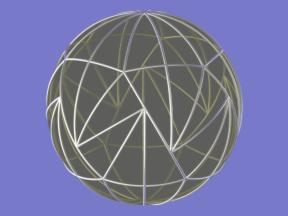Several right-angled tiles are mentioned above as being derived from isosceles tiles. This one is not, although both the 144°-30°-30° and 72°-72°-60° triangles can be seen in it. It can be thought of as a "rosette-and-filler" tiling like many others, but the rosettes are unusually large and ornate. It, and the 120°-45°-30° tile above, are also noteworthy for having four different types of vertex. This means that the vertex angles satisfy three simultaneous linear equations beyond the one that says how many triangles meet at the pole. Thus, even without that datum, the angles are fully determined, so this is the only tiling of its kind. The 108° -90° -54° tile and its 90° -60° -54° subtile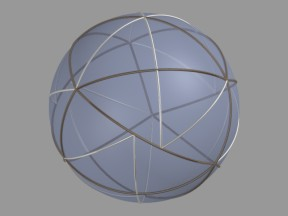This picture shows the 90° -60° -54° triangle, 30 copies of which tile the sphere. My wife noticed (thanks, Bridget!) that there were a lot of places in which three triangles fit together into a larger triangle (108° -90° -54° ). Upon investigation, it turned out that the entire tiling can be so divided, yielding a tiling with ten triangles. In fact, there are three of these, depending on how the top 108° -108° -108° triangle is divided up. Two of these are totally asymmetric, and the third has a symmetry group consisting of one 108° rotation. This is the smallest maximal symmetry group yet found for a tile, and suggests the question: Is there a tile that can tile the sphere - but only asymmetrically? It is also noteworthy that the 90° -60° -54° tiling is 2-colorable, like the 60° -60° -100° tiling. It has four sorts of vertex, each of which has four or six tiles meeting at a point. The 105° -90° -45° tile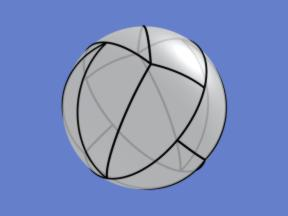This tiles only in one way, and is chiefly noteworthy for having a right angle and an obtuse angle, like the larger tile in the previous picture. The 90°-75°-45° tile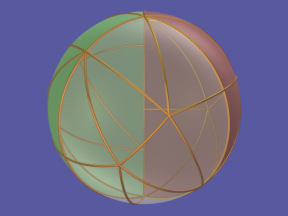Eight copies of this unusual tile tile a 120° lune; and three of those lunes tile the sphere. However, the tiling of the lune has chiral symmetry and not mirror symmetry, so that there must be at least one meridian along which the edge do not match up (visible here at the back, through the translucent front lune.) Except on this meridian, however,the tiling is edge-to-edge; and it would give an edge-to-edge double cover. This tiling has some interesting vertex equations, notably 3b + 3c at the poles. This equation cannot occur in an edge-to-edge tiling, so that - despite its "near" edge-to-edge nature, it was quickly eliminated by Sommerville and by Davies. There is a second tiling in which the three lunes all have the same handedness, which has a symmetry group of order 6. The 90° -78.75° -33.75° tile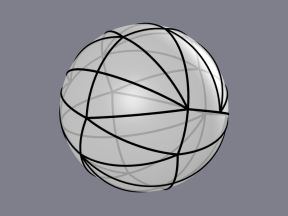This triangle, with angles of 90°, 78.75°, and 33.75° , was discovered by Blair Doyle, an undergraduate student at Saint Mary's. Blair was working with me to finish the classification of right triangles that tile the sphere and, almost at the end of the list of possible tiles, found this beauty. Its exceptionally irregular tiling pattern is slightly reminiscent of one of the Sommerville-Davies tilings, but gnarlier. (June 13, 2001) This tile probably completes the list of right triangles that tile the sphere; these are (as far as we know): the infinite family of right semilunes(90°-90°-360°/n) the infinite family of quarterlunes (edge-to-edge if the polar angle is 360° /2n) the continuum family of semilunes with 90° polar angle; the (90°-67.5°-67.5°) triangle, which is the n=4 case of a family that otherwise have no right angles the edge-to-edge (90°-60°-60°) tile (barycentric subdivision of the spherical tetrahedron) the edge-to-edge (90°-60°-45°) tile (barycentric subdivision of the spherical octahedron) the edge-to-edge (90°-60°-36°) tile (barycentric subdivision of the spherical icosahedron) the (90°-75°-60°) tile obtained by bisecting the (150°-60°-60°) tile the (90°-60°-50°) tile obtained by bisecting the (100°-60°-60°) tile the (90°-60°-40°) tile obtained by bisecting the (80°-60°-60°) tile or as a quarter of the (120°-60°-40°) tile. the (90°-108°-54°) tile and its (90°-60°-54°) subtile the (90°-105°-45°) tile the (90°-75°-45°) tile the (90°-72°-30°) tile this tile. This list will be confirmed complete or updated soon. A near tiling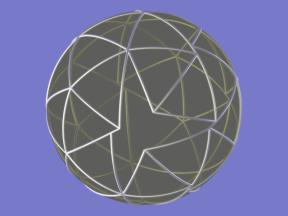It can be proved that the 75°-60°-60° triangle cannot tile the sphere. However, it does permit a five-fold tiling of the sphere; and it comes very close to a single tiling. This image shows an exact partial tiling with 46 tiles (the area of one tile is exactly 1/48 that of the sphere). I conjecture that this is the best that we can do. It can be seen that there are three complete layers of tiles around one [in back, just above the center] tile, so this tile has Heesch number 3. Is this a record for the sphere? A puzzle using the 90°-60°-40° tile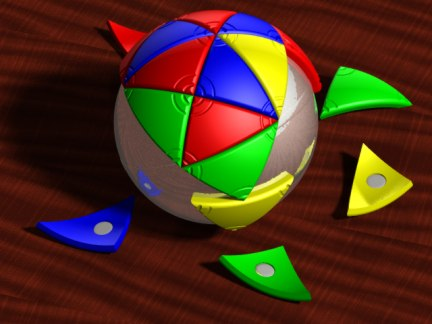This is a "fiddling" sort of puzzle like Rubik's Snake or Tangrams: solving it is easy, the challenge is in seeing how many patterns you can get! Three tilings appear as subdivisions of tilings with the 80°-60°-60° triangle, but there are others. The puzzle has never actually been built, though the ray-tracing has fooled a couple people. Blair Doyle and I looked into patenting it but decided against it. If you want to manufacture these, feel free - just give us credit in the instruction sheet and send us a couple! You will need to provide a minimum of 72 tiles, but different tilings require a different mix of left- and right-handed tiles. One approach would be to provide some extras; another would be to determine what the maximum imbalance could be and make the puzzle harder by providing a set of tiles that can only tile in one (or a few?) ways. The pictures above were generated using POV-Ray. An article about some of these tilings is available online.